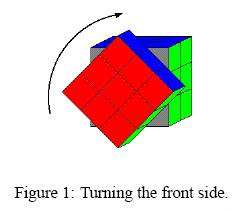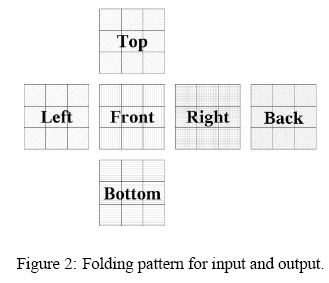Online JudgeProblem SetAuthorsOnline ContestsUser
Web Board
F.A.Qs
Statistical Charts
Problems
Submit Problem
Online Status
Prob.ID:
Register
Authors ranklist
Current Contest
Past Contests
Scheduled Contests
Award Contest
Register
Language:
Rubik's Cube
 Time Limit: 1000MS Memory Limit: 30000K Total Submissions: 789 Accepted: 367

Description

Background
Rummaging through the stuff of your childhood you find an old toy which you identify as the famous Rubik's Cube. While playing around with it you have to acknowledge that throughout the years your ability to solve the puzzle has not improved a bit. But because you always wanted to understand the thing and the only other thing you could do right now is to prepare for an exam, you decide to give it a try. Luckily the brother of your girlfriend is an expert and able to fix the cube no matter how messed-up it is. The problem is that he stays with his girlfriend in the Netherlands most of the time, so you need a solution for long-distance learning. You decide to implement a program which is able to document the state of the cube and the turns to be made.
Problem
A Rubik's Cube is covered with 54 square areas called facelets, 9 facelets on each of its six sides. Each facelet has a certain color. Usually when the cube is in its starting state, all facelets belonging to one side have the same color. For the original cube these are red, yellow, green, blue, white and orange.The positions of the facelets can be changed by turning the sides of the cube. This moves nine "little cubes" together with their attached facelets into a new position (see Fig. 1).
The problem is to determine how the facelets of the entire cube are colored after turning different sides in different directions.

Input

The first line contains the number of scenarios. Each scenario consists of two sections. The first section describes the starting state of the cube and the second describes the turns to be made.
The starting state describes the colors of the facelets and where they are positioned. The colors are identified by single characters, and one character is given per facelet. Characters are separated by blanks and arranged in a certain pattern (see Fig. 2). The pattern identifies all six sides of the cube and can be thought of as a folding pattern. As shown in Fig. 2, the description of the top side of the cube is placed right over the description of the front side. This is done by indenting the lines with blanks. The next three lines contain the descriptions of the left, front, right and back side as shown in Fig. 2. The descriptions are simply concatenated with a blank character used as separator. After that the description of the bottom side follows, using the same format as the one used to describe the top side. This concludes the description of the starting state.
Then follows the second section of the scenario containing the turns which have to be performed. The description of the turns starts with a line containing the number of turns t (t > 0). Each turn is given in a separate line and consists of two integer values s and d which are separated by a single blank. The first value s determines the side of the cube which has to be turned. The sides are serially numbered as follows:left '0', front '1', right '2', back '3', top '4', bottom '5'. The second value d determines in which directionthe side s has to be turned and can either be '1' or '-1'. A '1' stands for clockwise and a '-1' for counterclockwise.The direction is given under the assumption that the viewer is looking directly at the specific side of the cube.

Output

The output for every scenario begins with a line containing "Scenario #i:", where i is the number of the scenario starting at 1. After this line print the resulting state of the cube using the same format as the input. Each scenario is terminated by a single blank line.

Sample Input

```2
w w w
w w w
w w w
r r r g g g b b b o o o
r r r g g g b b b o o o
r r r g g g b b b o o o
y y y
y y y
y y y
2
3 1
0 -1
g b b
g w w
g w w
r r r y g g b b y o o w
r r r y g g b b y o o w
w w w r g g b b y o o b
o y y
o y y
o r r
2
0 1
3 -1
```

Sample Output

```Scenario #1:
g b b
g w w
g w w
r r r y g g b b y o o w
r r r y g g b b y o o w
w w w r g g b b y o o b
o y y
o y y
o r r

Scenario #2:
w w w
w w w
w w w
r r r g g g b b b o o o
r r r g g g b b b o o o
r r r g g g b b b o o o
y y y
y y y
y y y
```

Source

TUD Programming Contest 2002, Darmstadt, Germany

[Submit]   [Go Back]   [Status]   [Discuss]Home PageGo BackTo top